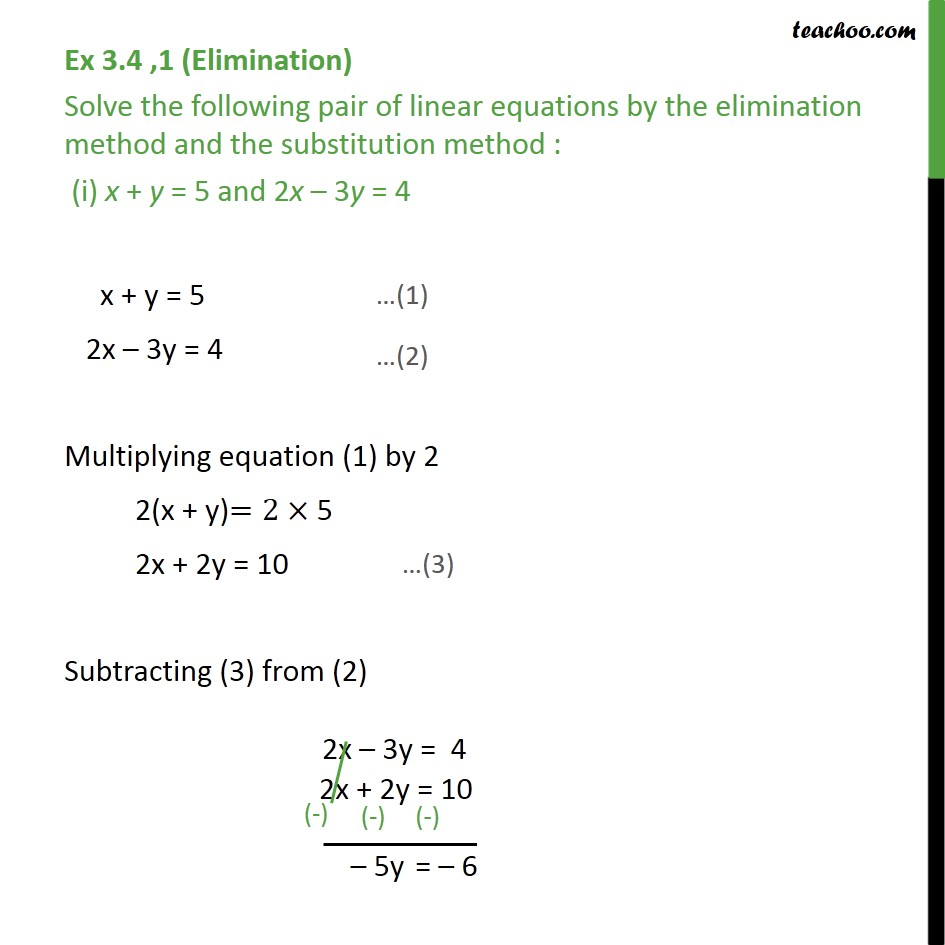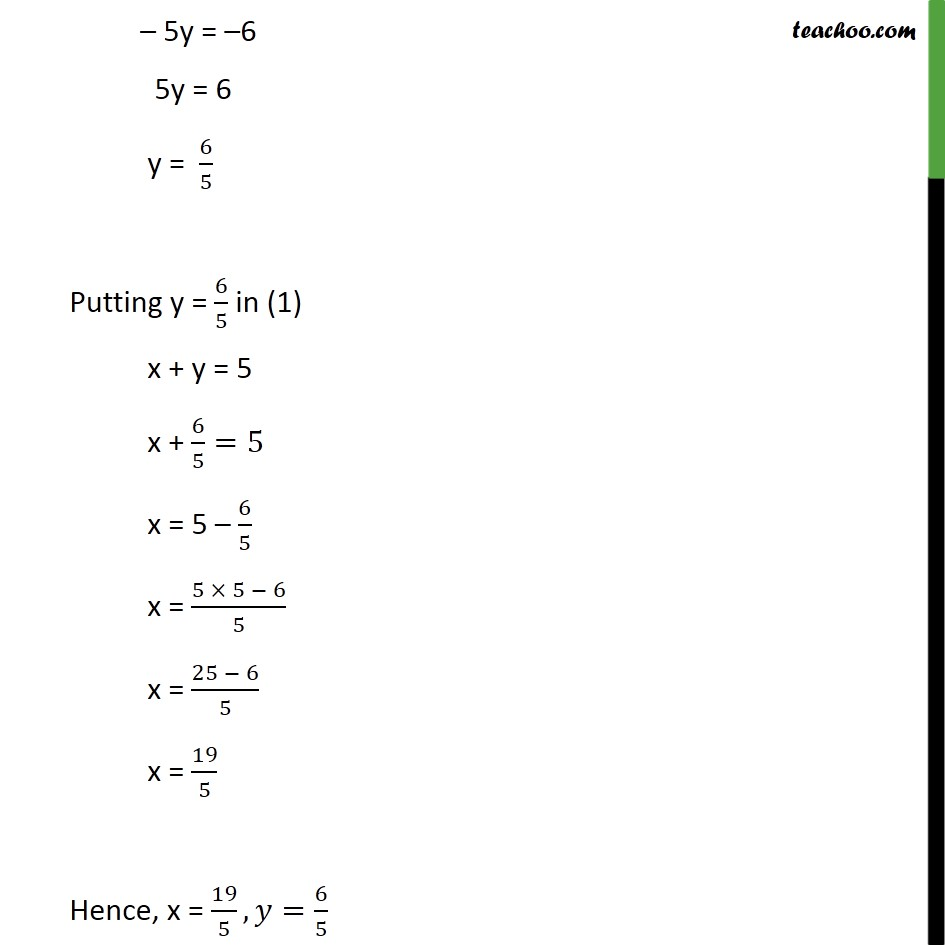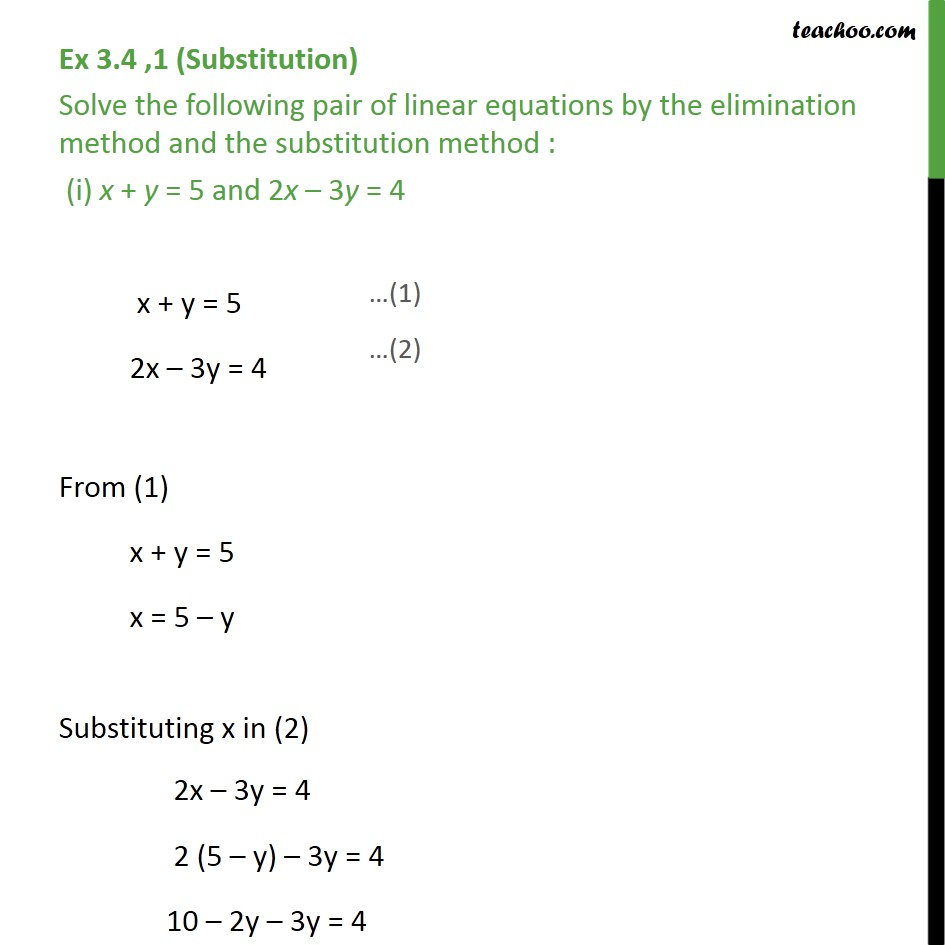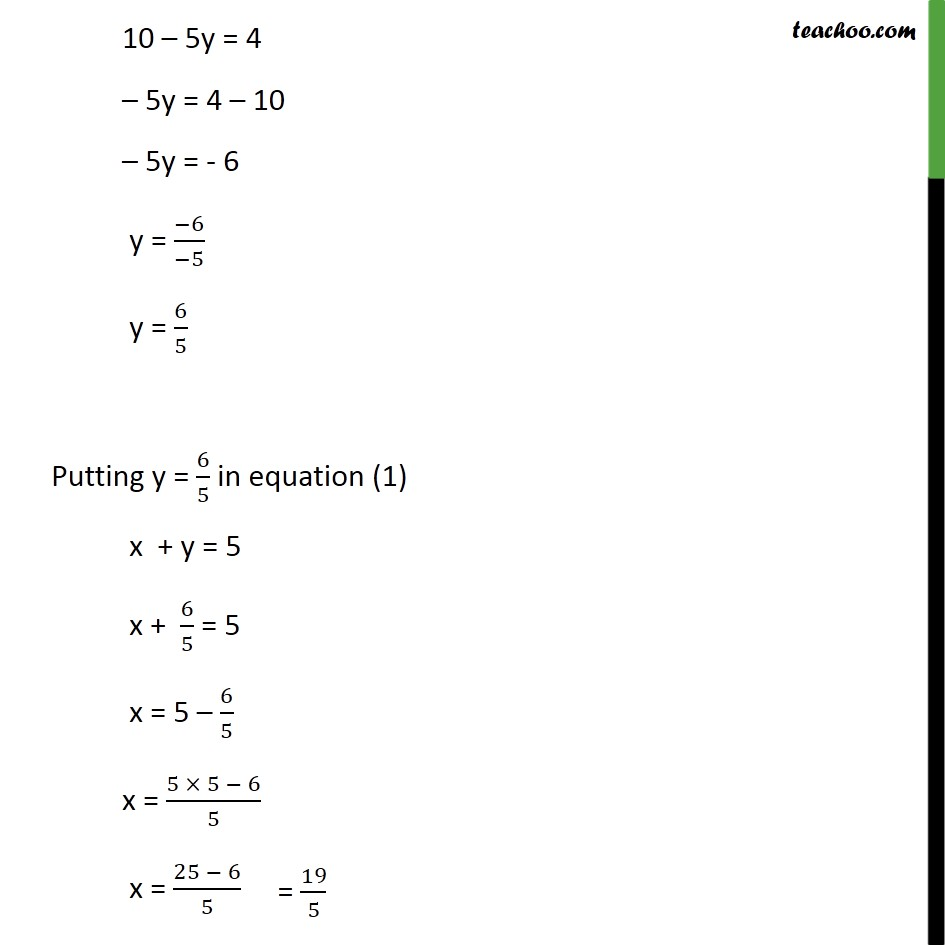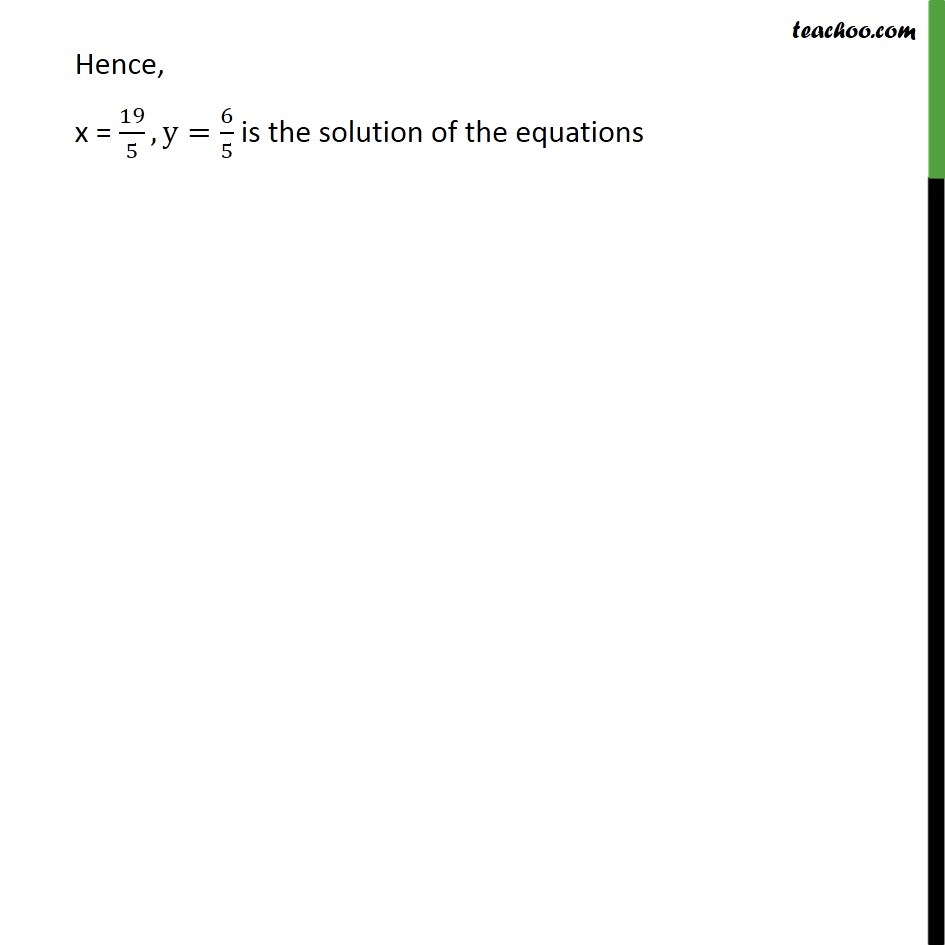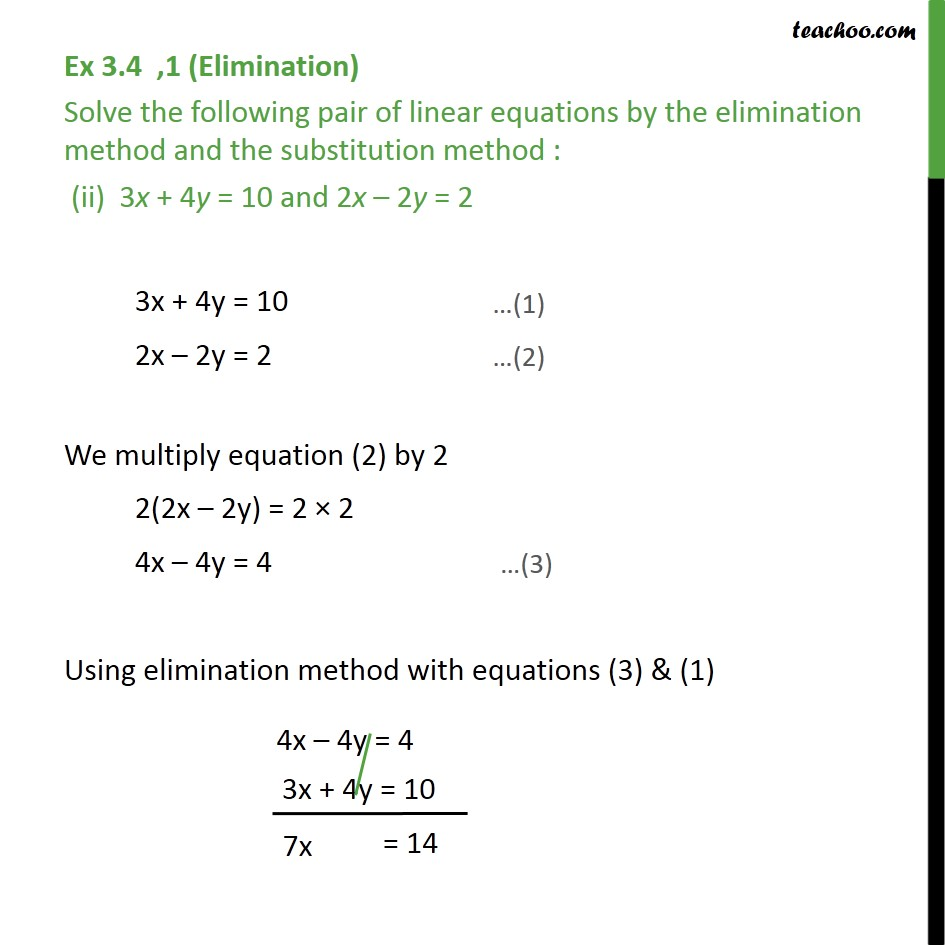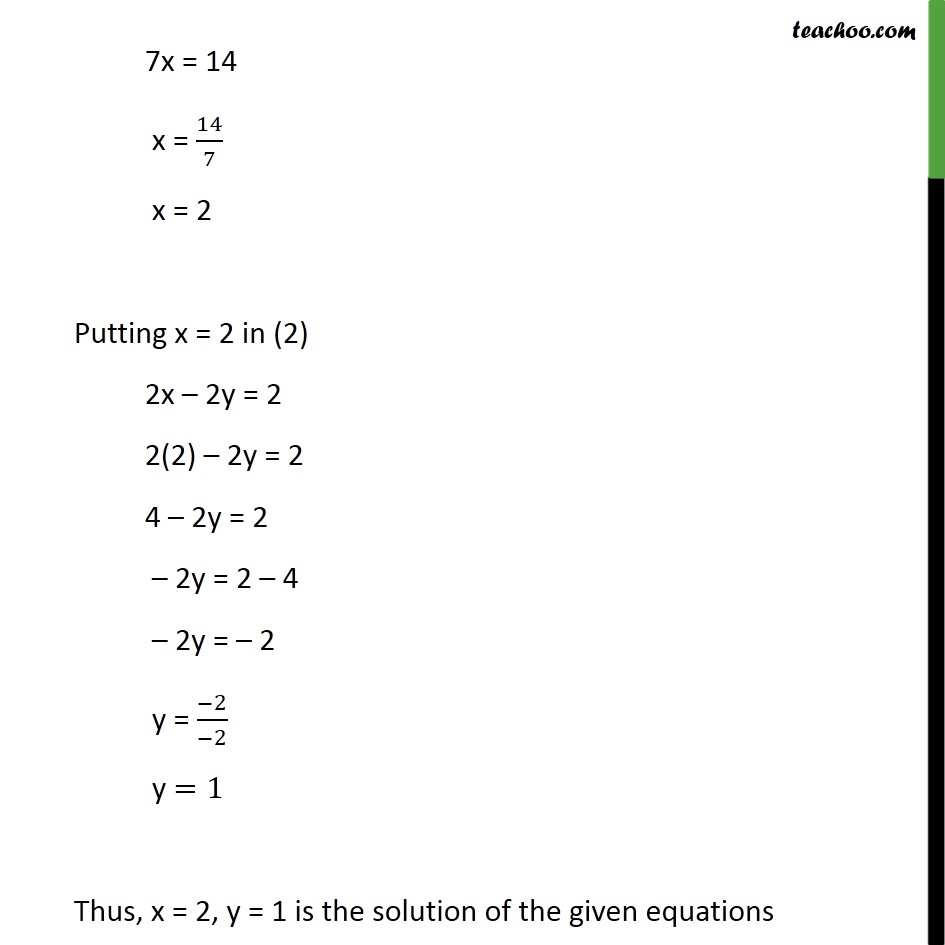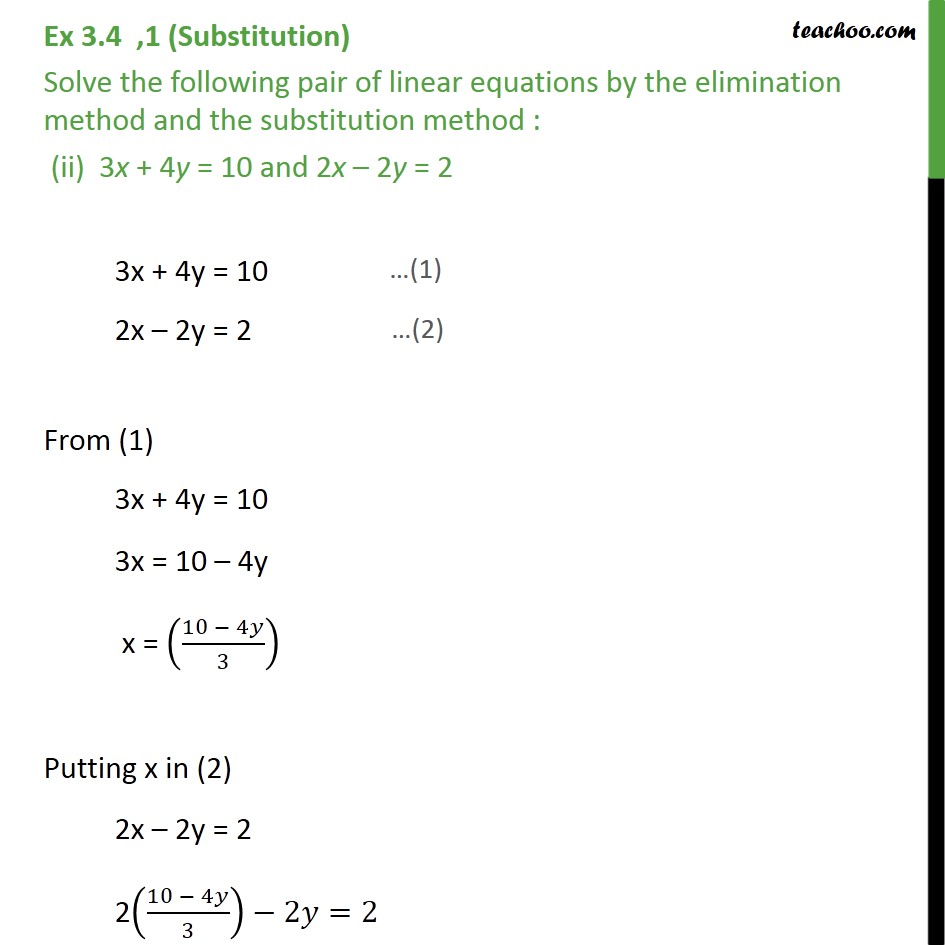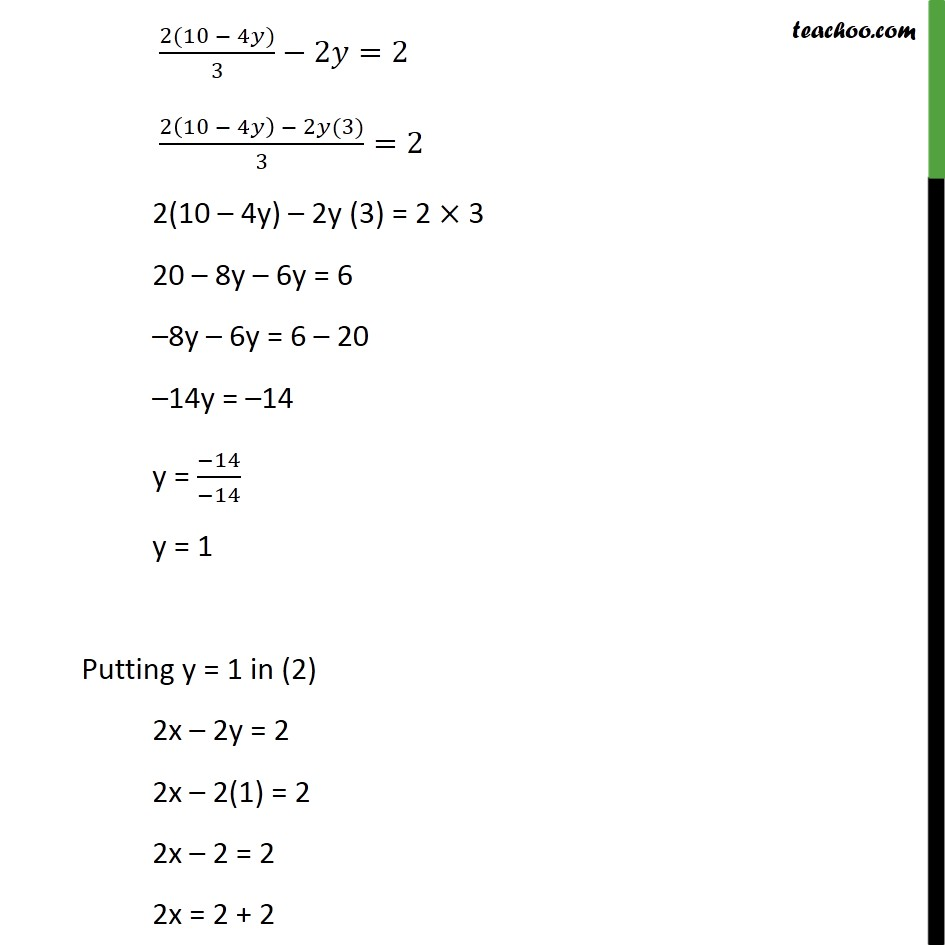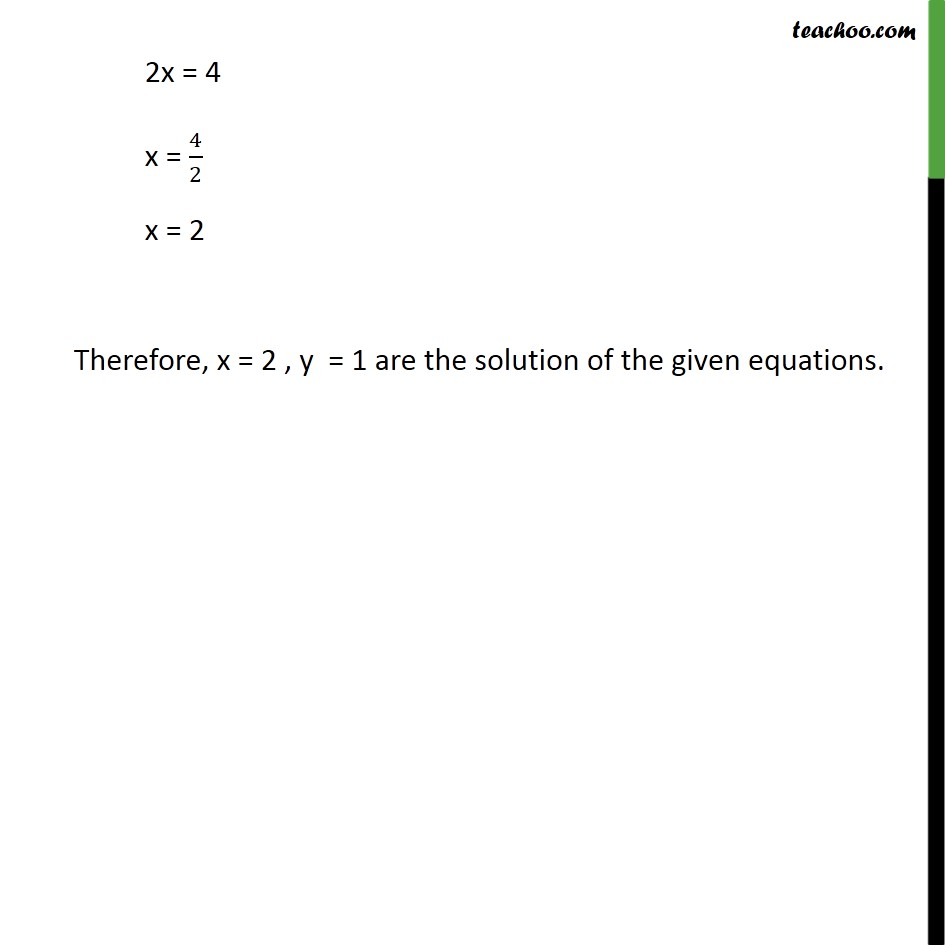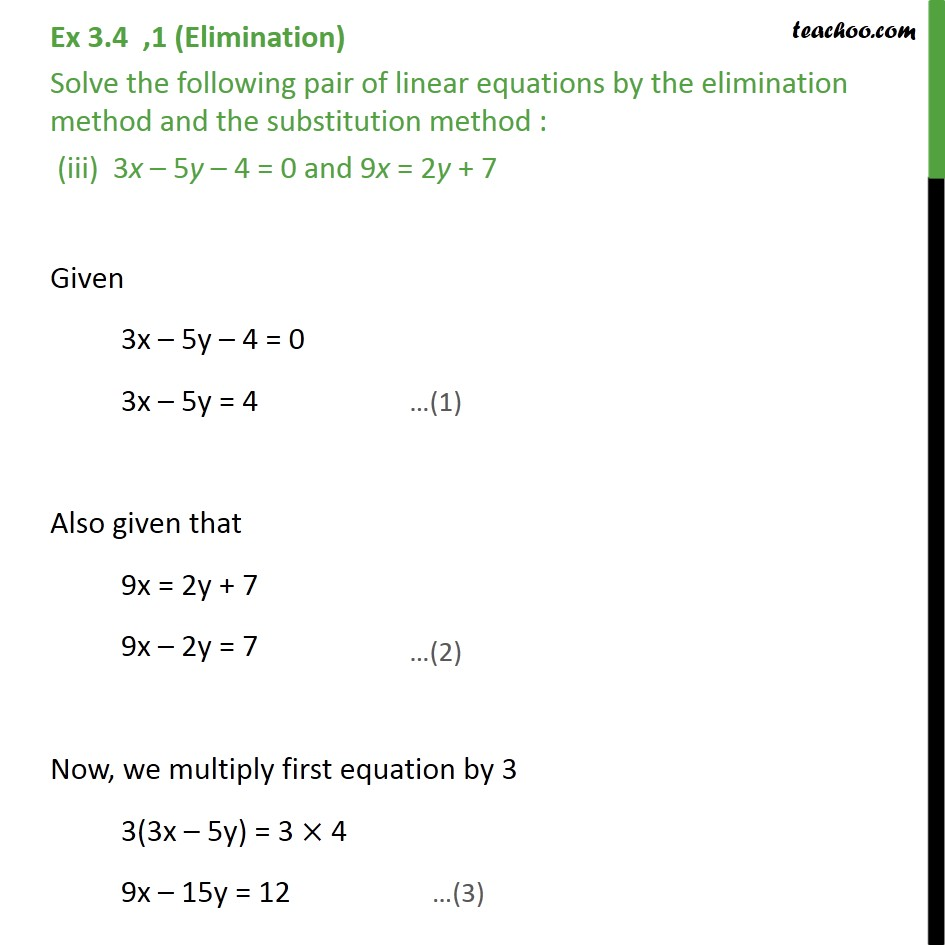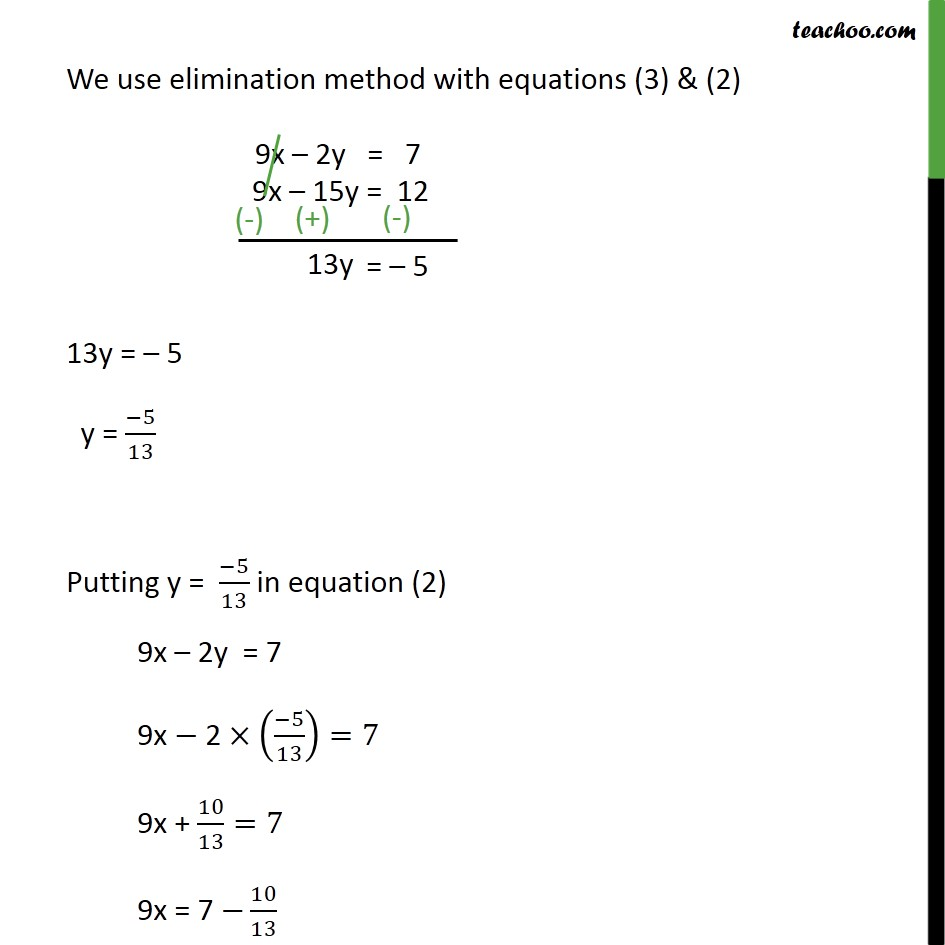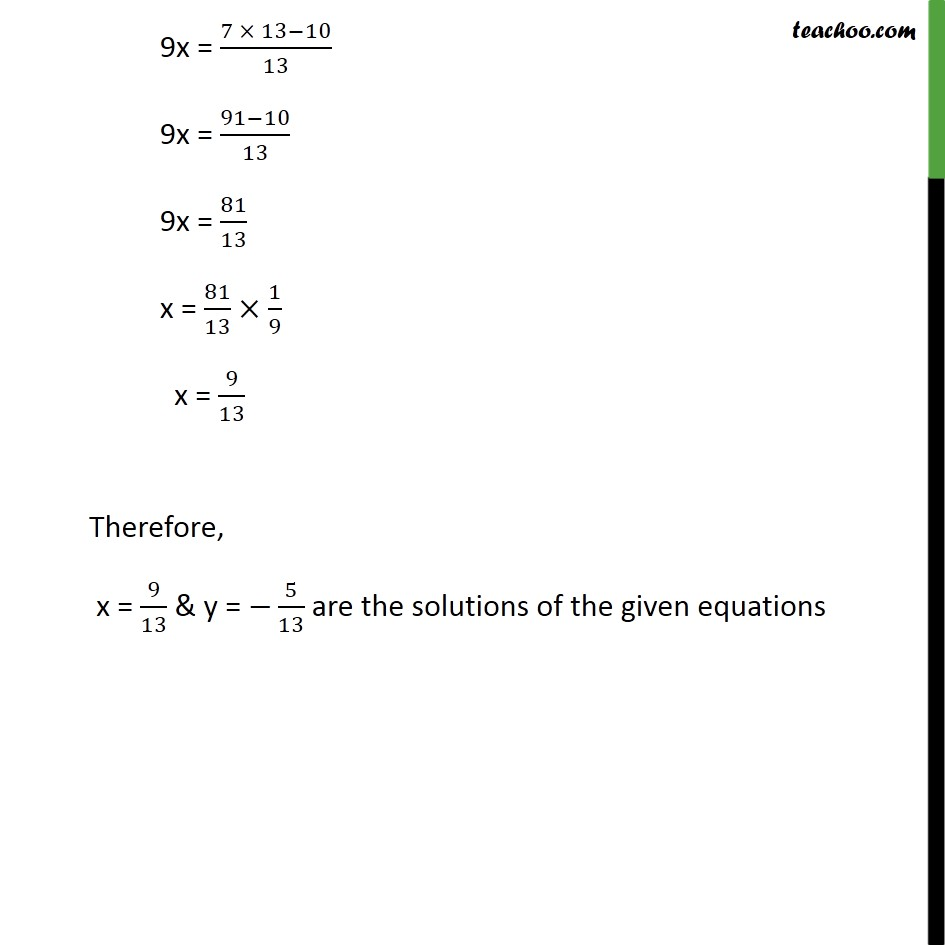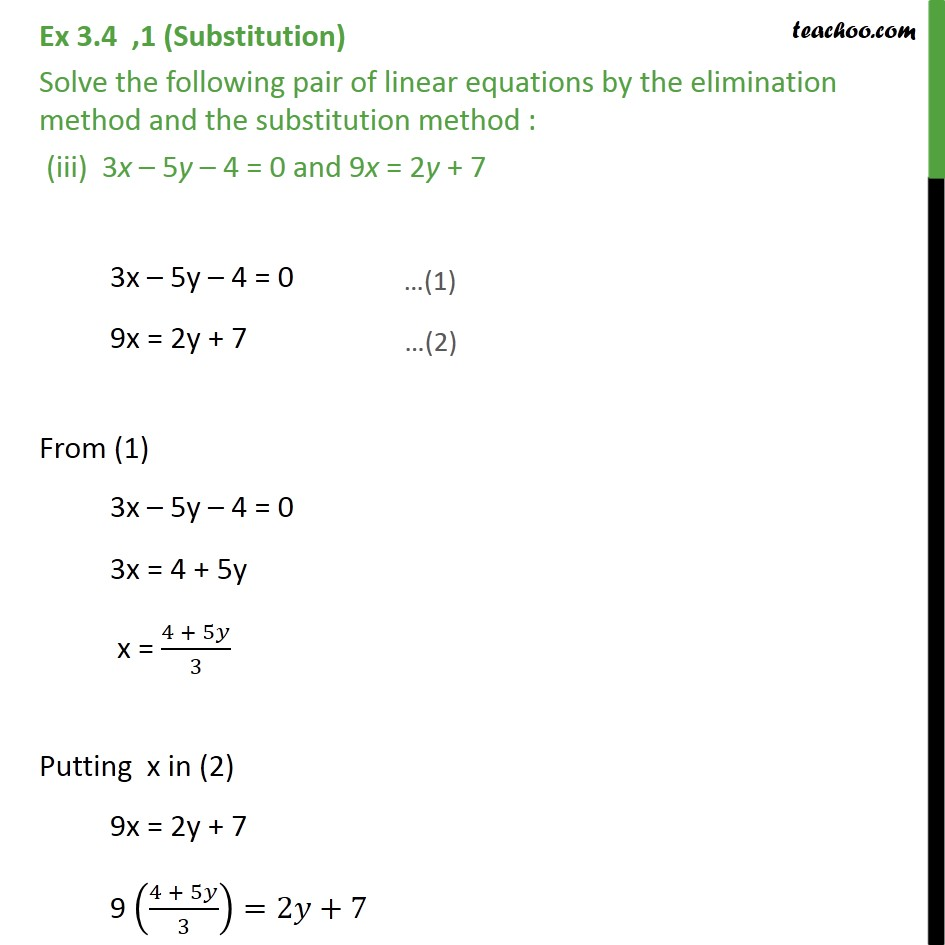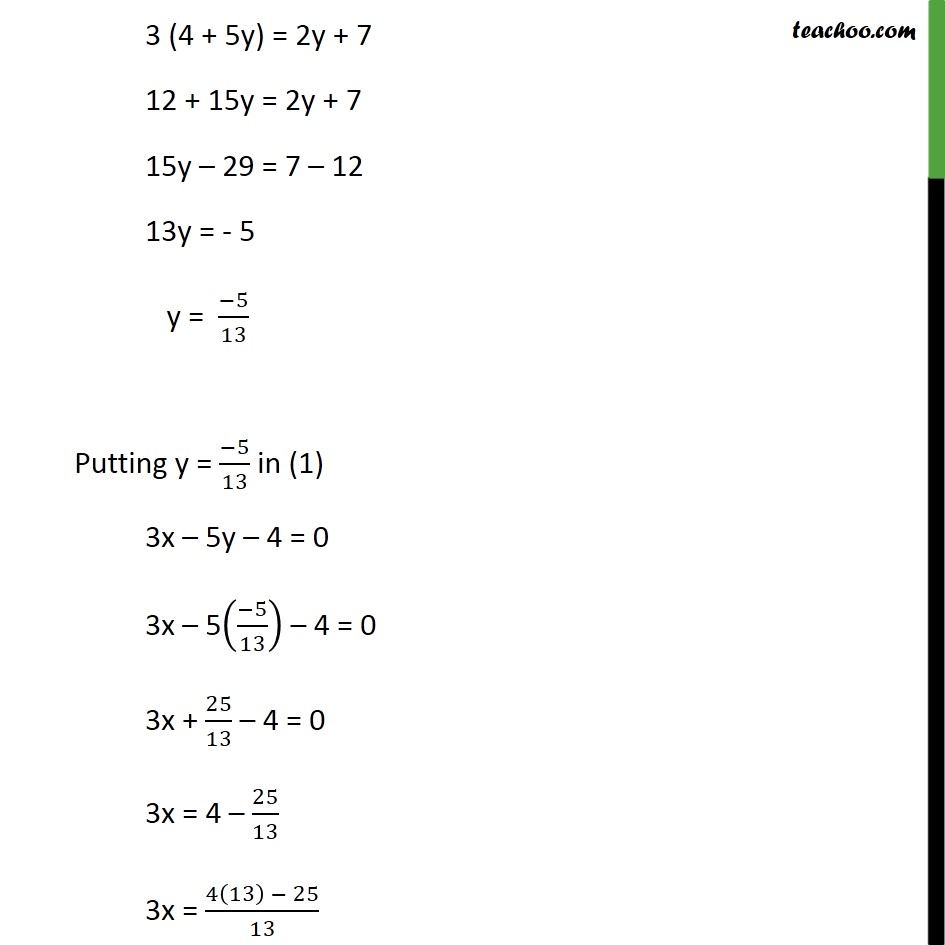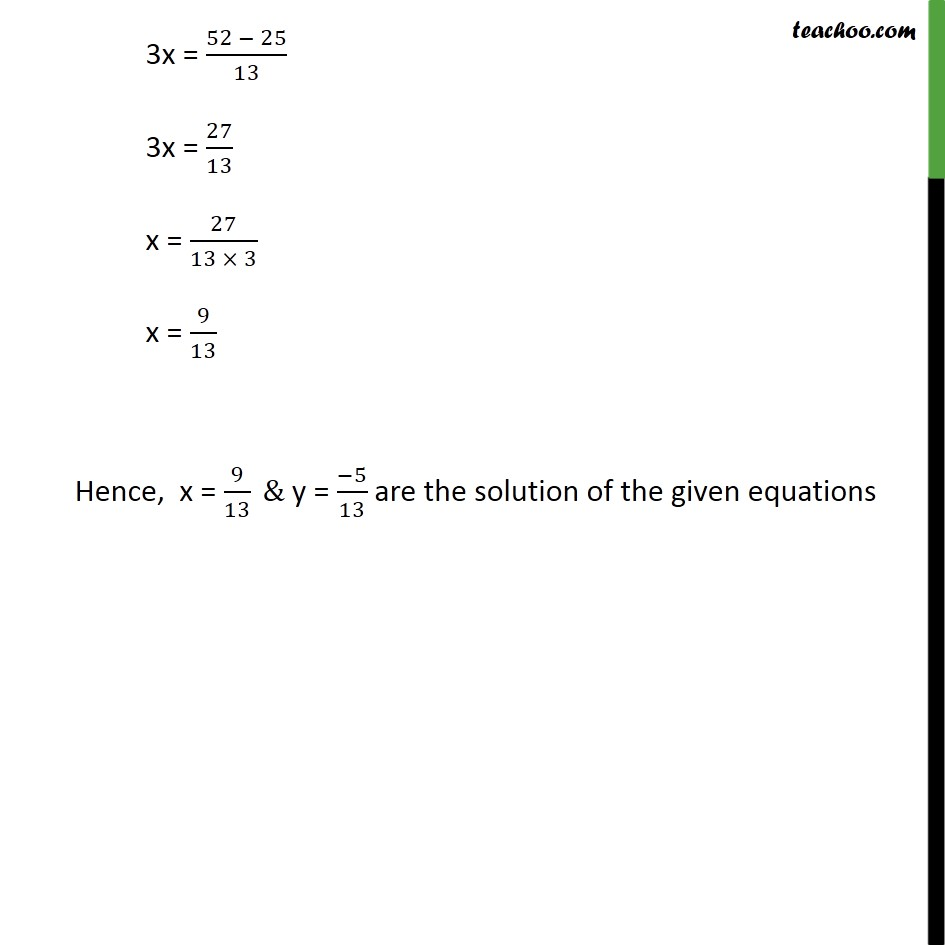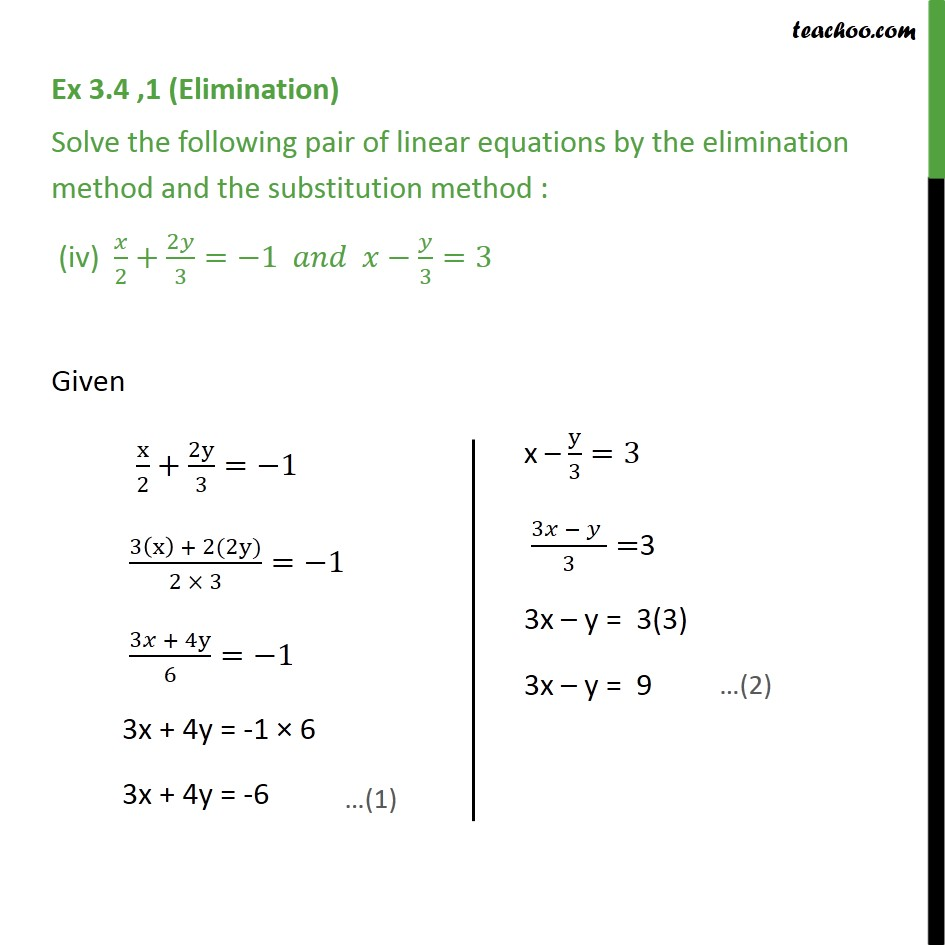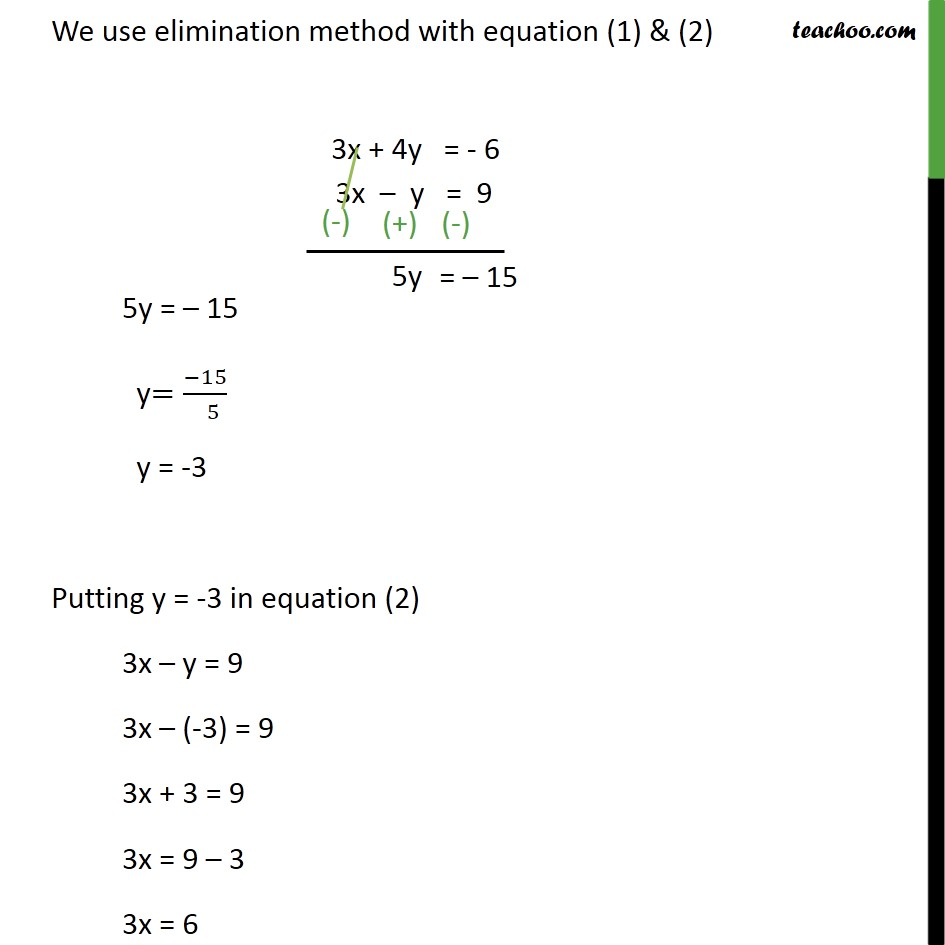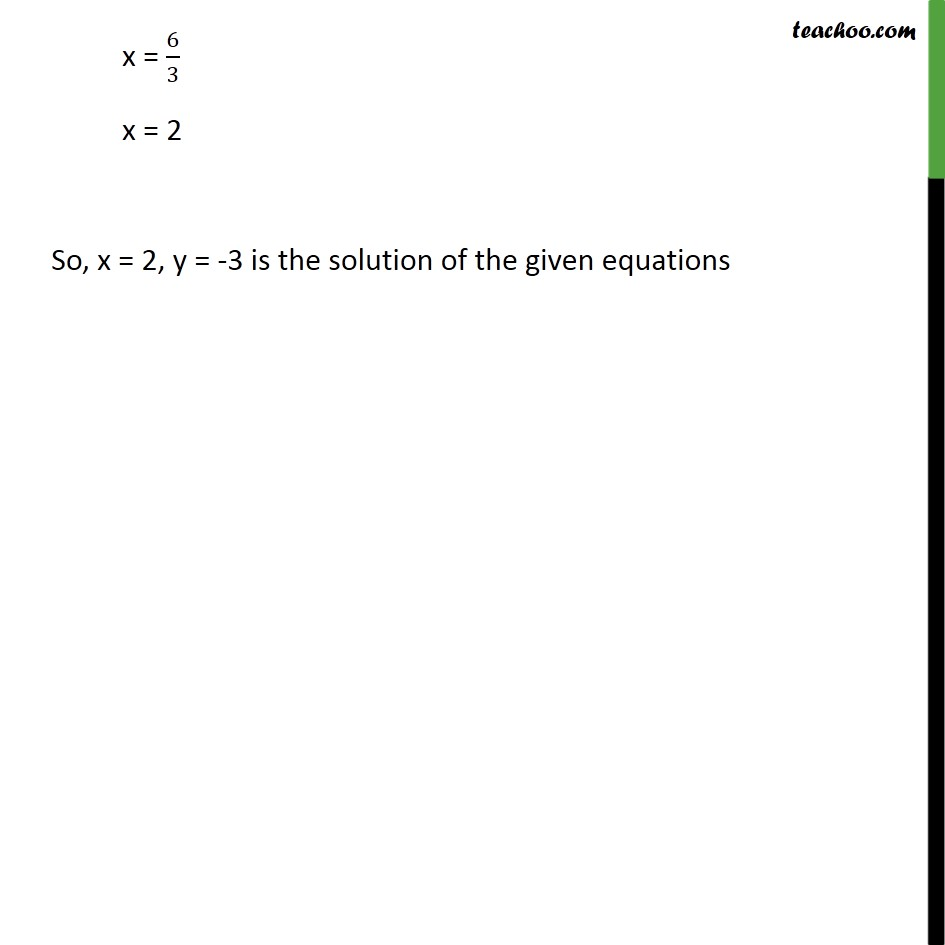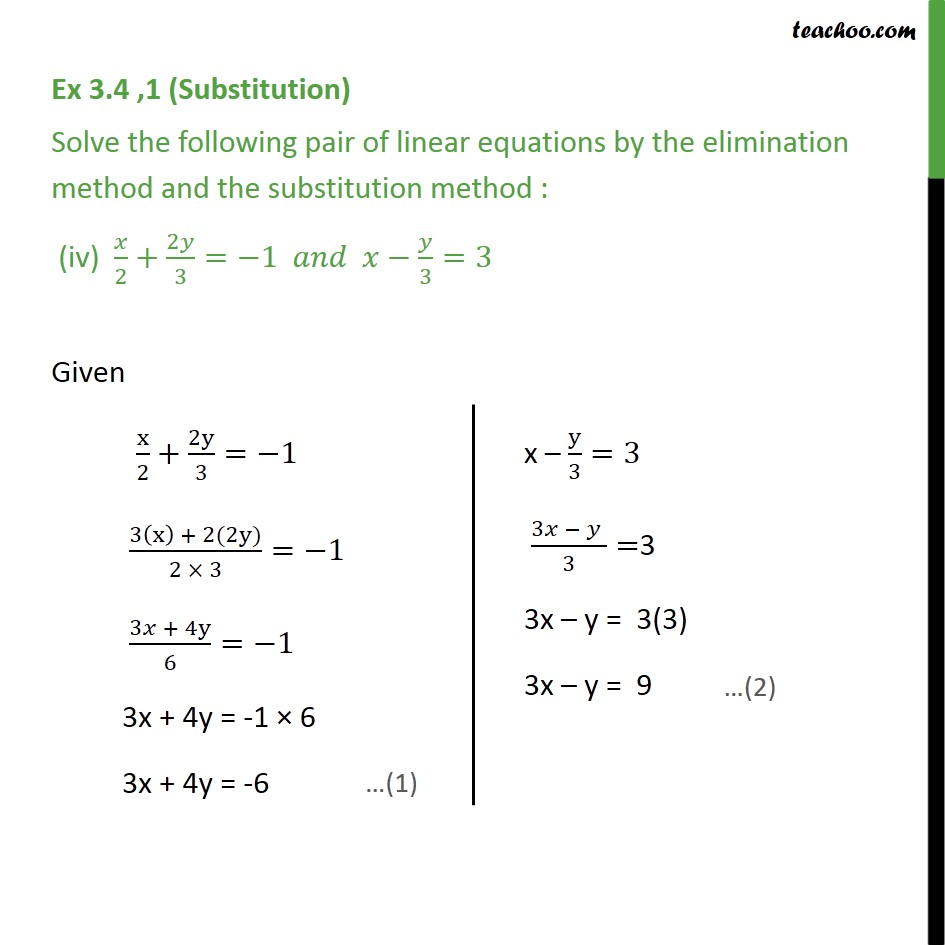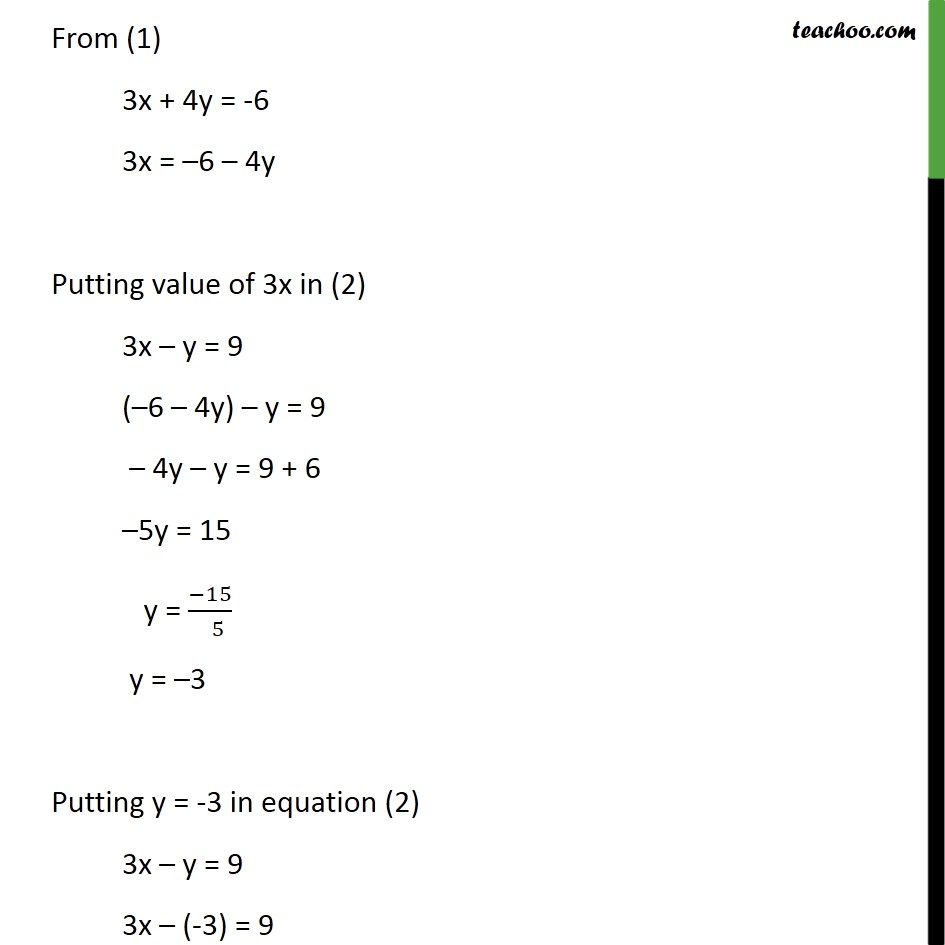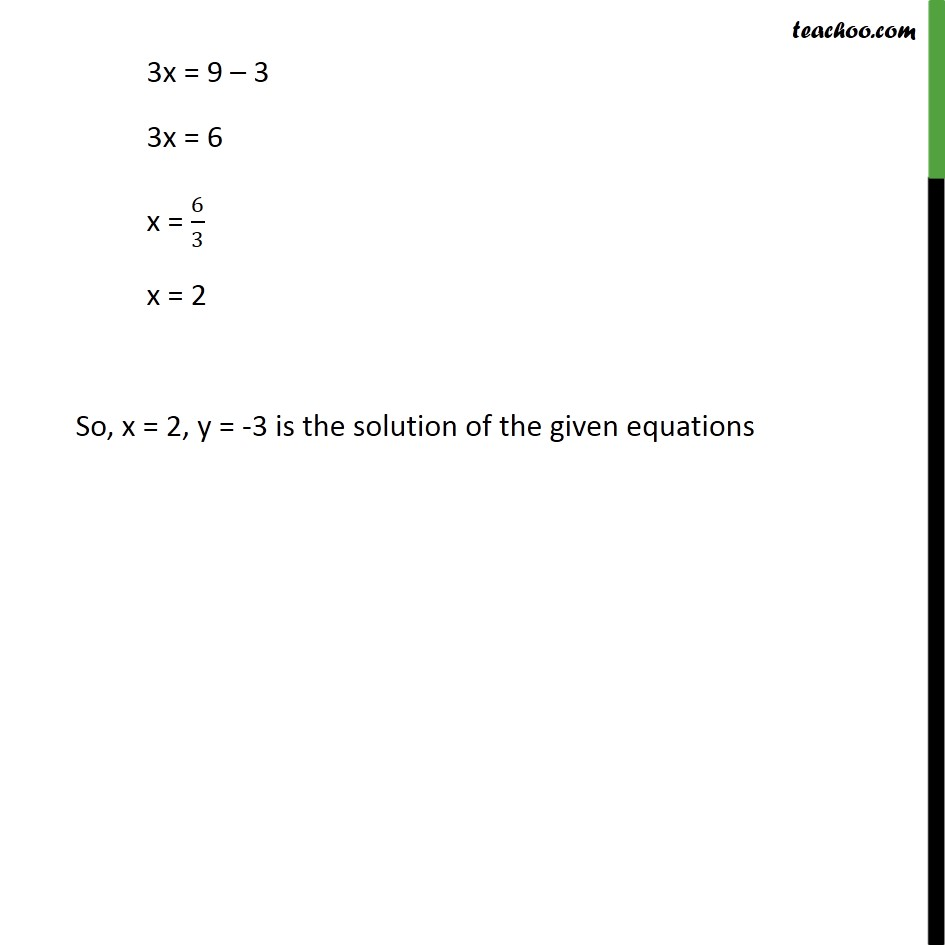1. Chapter 3 Class 10 Pair of Linear Equations in Two Variables
2. Serial order wise
3. Ex 3.4

Transcript

Ex 3.4 ,1 (Elimination) Solve the following pair of linear equations by the elimination method and the substitution method : (i) x + y = 5 and 2x – 3y = 4 x + y = 5 2x – 3y = 4 Multiplying equation (1) by 2 2(x + y)="2"× 5 2x + 2y = 10 Subtracting (3) from (2) – 5y = –6 5y = 6 y = 6/5 Putting y = 6/5 in (1) x + y = 5 x + 6/5=5 x = 5 – 6/5 x = (5 × 5 − 6)/5 x = (25 − 6)/5 x = 19/5 Hence, x = 19/5,𝑦=6/5 Ex 3.4 ,1 (Substitution) Solve the following pair of linear equations by the elimination method and the substitution method : (i) x + y = 5 and 2x – 3y = 4 x + y = 5 2x – 3y = 4 From (1) x + y = 5 x = 5 – y Substituting x in (2) 2x – 3y = 4 2 (5 – y) – 3y = 4 10 – 2y – 3y = 4 10 – 5y = 4 – 5y = 4 – 10 – 5y = - 6 y = (−6)/(−5) y = 6/5 Putting y = 6/5 in equation (1) x + y = 5 x + 6/5 = 5 x = 5 – 6/5 x = (5 × 5 − 6)/5 x = (25 − 6)/5 Hence, x = 19/5,y=6/5 is the solution of the equations Ex 3.4 ,1 (Elimination) Solve the following pair of linear equations by the elimination method and the substitution method : (ii) 3x + 4y = 10 and 2x – 2y = 2 3x + 4y = 10 2x – 2y = 2 We multiply equation (2) by 2 2(2x – 2y) = 2 × 2 4x – 4y = 4 Using elimination method with equations (3) & (1) 7x = 14 x = 14/7 x = 2 Putting x = 2 in (2) 2x – 2y = 2 2(2) – 2y = 2 4 – 2y = 2 – 2y = 2 – 4 – 2y = – 2 y = (−2)/(−2) y =1 Thus, x = 2, y = 1 is the solution of the given equations Ex 3.4 ,1 (Substitution) Solve the following pair of linear equations by the elimination method and the substitution method : (ii) 3x + 4y = 10 and 2x – 2y = 2 3x + 4y = 10 2x – 2y = 2 From (1) 3x + 4y = 10 3x = 10 – 4y x = ((10 − 4𝑦)/3) Putting x in (2) 2x – 2y = 2 2((10 − 4𝑦)/3)−2𝑦=2 (2(10 − 4𝑦))/3−2𝑦=2 (2(10 − 4𝑦) − 2𝑦(3))/3=2 2(10 – 4y) – 2y (3) = 2 × 3 20 – 8y – 6y = 6 –8y – 6y = 6 – 20 –14y = –14 y = (−14)/(−14) y = 1 Putting y = 1 in (2) 2x – 2y = 2 2x – 2(1) = 2 2x – 2 = 2 2x = 2 + 2 2x = 4 x = 4/2 x = 2 Therefore, x = 2 , y = 1 are the solution of the given equations. Ex 3.4 ,1 (Elimination) Solve the following pair of linear equations by the elimination method and the substitution method : (iii) 3x – 5y – 4 = 0 and 9x = 2y + 7 Given 3x – 5y – 4 = 0 3x – 5y = 4 Also given that 9x = 2y + 7 9x – 2y = 7 Now, we multiply first equation by 3 3(3x – 5y) = 3 × 4 9x – 15y = 12 We use elimination method with equations (3) & (2) 13y = – 5 y = (−5)/13 Putting y = (−5)/13 in equation (2) 9x – 2y = 7 9x −" 2"×((−5)/13)=7 9x + 10/13=7 9x = 7 −10/13 9x = (7 × 13−10)/13 9x = (91−10)/13 9x = 81/13 x = 81/13×1/9 x = 9/13 Therefore, x = 9/13 & y = −5/13 are the solutions of the given equations Ex 3.4 ,1 (Substitution) Solve the following pair of linear equations by the elimination method and the substitution method : (iii) 3x – 5y – 4 = 0 and 9x = 2y + 7 3x – 5y – 4 = 0 9x = 2y + 7 From (1) 3x – 5y – 4 = 0 3x = 4 + 5y x = (4 + 5𝑦)/3 Putting x in (2) 9x = 2y + 7 9 ((4 + 5𝑦)/3)=2𝑦+7 3 (4 + 5y) = 2y + 7 12 + 15y = 2y + 7 15y – 29 = 7 – 12 13y = - 5 y = (−5)/13 Putting y = (−5)/13 in (1) 3x – 5y – 4 = 0 3x – 5((−5)/13) – 4 = 0 3x + 25/13 – 4 = 0 3x = 4 – 25/13 3x = (4(13) − 25)/13 3x = (52 − 25)/13 3x = 27/13 x = 27/(13 × 3) x = 9/13 Hence, x = 9/13 & y = (−5)/13 are the solution of the given equations Ex 3.4 ,1 (Elimination) Solve the following pair of linear equations by the elimination method and the substitution method : (iv) 𝑥/2+2𝑦/3=−1 𝑎𝑛𝑑 𝑥−𝑦/3=3 Given x/2+2y/3=−1 (3(x) + 2(2y))/(2 × 3)=−1 (3𝑥 + 4y)/6=−1 3x + 4y = -1 × 6 3x + 4y = -6 We use elimination method with equation (1) & (2) 5y = – 15 y=(−15)/( 5) y = -3 Putting y = -3 in equation (2) 3x – y = 9 3x – (-3) = 9 3x + 3 = 9 3x = 9 – 3 3x = 6 x = 6/3 x = 2 So, x = 2, y = -3 is the solution of the given equations Ex 3.4 ,1 (Substitution) Solve the following pair of linear equations by the elimination method and the substitution method : (iv) 𝑥/2+2𝑦/3=−1 𝑎𝑛𝑑 𝑥−𝑦/3=3 Given x/2+2y/3=−1 (3(x) + 2(2y))/(2 × 3)=−1 (3𝑥 + 4y)/6=−1 3x + 4y = -1 × 6 3x + 4y = -6 From (1) 3x + 4y = -6 3x = –6 – 4y Putting value of 3x in (2) 3x – y = 9 (–6 – 4y) – y = 9 – 4y – y = 9 + 6 –5y = 15 y = (−15)/( 5) y = –3 Putting y = -3 in equation (2) 3x – y = 9 3x – (-3) = 9 3x = 9 – 3 3x = 6 x = 6/3 x = 2 So, x = 2, y = -3 is the solution of the given equations

Ex 3.4